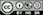Estimate a Neural Network

To estimate a model select the type (i.e., Classification or Regression), response variable, and one or more explanatory variables. Press the Estimate button or CTRL-enter (CMD-enter on mac) to generate results. The model can be “tuned” by changing the Size (i.e., the number of nodes in the hidden layer) and by adjusting the Decay rate. The higher the value set for Decay, the higher the penalty on the size of (the sum of squares of) the weights. When Decay is set to 0, the model has the most flexibility to fit the (training) data accurately. However, without Decay the model is also more likely to overfit.

The best way to determine the optimal values for Size and Decays is to use Cross-Validation. In radiant, you can use the cv.nn function for this purpose. See the documentation for more information.

### Report > Rmd

Add code to Report > Rmd to (re)create the analysis by clicking the icon on the bottom left of your screen or by pressing ALT-enter on your keyboard.

If either a Olden or Garson plot was created it can be customized using ggplot2 commands (e.g., plot(result, plots = "garson", custom = TRUE) + labs(title = "Garson plot")). See Data > Visualize for details.

To add, for example, a title to a network plot use title(main = "Network plot"). See the R graphics documentation for additional information.

### R-functions

For an overview of related R-functions used by Radiant to estimate a neural network model see Model > Neural network.

The key function from the nnet package used in the nn tool is nnet.

© Vincent Nijs (2019)